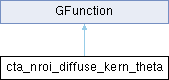GammaLib  1.7.0.dev
cta_nroi_diffuse_kern_theta Class Reference

Kernel for Nroi offest angle integration of diffuse model. More...

#include <GCTAResponse_helpers.hpp>

Inheritance diagram for cta_nroi_diffuse_kern_theta:## Public Member Functions

cta_nroi_diffuse_kern_theta (const GCTAResponseIrf *rsp, const GCTAObservation *obs, const GModelSpatial *model, const GMatrix *rot, const GEnergy &srcEng, const GTime &srcTime, const GEnergy &obsEng, const GTime &obsTime, const int &iter)

double eval (const double &theta)
Kernel for Nroi offest angle integration of diffuse model. More...Public Member Functions inherited from GFunction
GFunction (void)
Void constructor. More...

GFunction (const GFunction &function)
Copy constructor. More...

virtual ~GFunction (void)
Destructor. More...

GFunctionoperator= (const GFunction &function)
Assignment operator. More...

## Protected Attributes

const GCTAResponseIrfm_rsp
CTA response. More...

const GCTAObservationm_obs
CTA observation. More...

const GModelSpatialm_model
Spatial model. More...

const GMatrixm_rot
Rotation matrix. More...

GEnergy m_srcEng
True photon energy. More...

GTime m_srcTime
True photon arrival time. More...

GEnergy m_obsEng
Observed photon energy. More...

GTime m_obsTime
Observed photon arrival time. More...

int m_iter
Integration iterations. More...Protected Member Functions inherited from GFunction
void init_members (void)
Initialise class members. More...

void copy_members (const GFunction &function)
Copy class members. More...

void free_members (void)
Delete class members. More...

## Detailed Description

Kernel for Nroi offest angle integration of diffuse model.

This class implements the integration kernel $$K(\theta)$$ for the integration

$\int_{0}^{\theta_{\rm max}} K(\theta | E, t) d\theta$

of diffuse models. The eval() method computes

$K(\theta | E, t) = \sin \theta \times \int_{0}^{2\pi} S_{\rm p}(\theta, \phi | E, t) \, N_{\rm pred}(\theta, \phi) d\phi$

where

• $$S_{\rm p}(\theta, \phi | E, t)$$ is the diffuse model,
• $$N_{\rm pred}(\theta, \phi)$$ is the data space integral of the Instrument Response Function for a point spread function over the Region Of Interest in the reference frame of the diffuse source model
• $$\theta$$ is the distance from the ROI centre, and
• $$\phi$$ is the azimuth angle.

Definition at line 929 of file GCTAResponse_helpers.hpp.

## Constructor & Destructor Documentation

 cta_nroi_diffuse_kern_theta::cta_nroi_diffuse_kern_theta ( const GCTAResponseIrf * rsp, const GCTAObservation * obs, const GModelSpatial * model, const GMatrix * rot, const GEnergy & srcEng, const GTime & srcTime, const GEnergy & obsEng, const GTime & obsTime, const int & iter )
inline

Definition at line 931 of file GCTAResponse_helpers.hpp.

## Member Function Documentation

 double cta_nroi_diffuse_kern_theta::eval ( const double & theta )
virtual

Kernel for Nroi offest angle integration of diffuse model.

Parameters
 [in] theta Offset angle with respect to ROI centre (radians).

Computes

$K(\theta | E, t) = \sin \theta \times \int_{0}^{2\pi} S_{\rm p}(\theta, \phi | E, t) \, N_{\rm pred}(\theta, \phi) d\phi$

This method integrates a diffuse model for a given offset angle with respect to the ROI centre over all azimuth angles (from 0 to 2pi). The integration is only performed for positive offset angles, otherwise 0 is returned.

Integration is done using the Romberg algorithm. The integration kernel is defined by the helper class cta_npred_diffuse_kern_phi.

Note that the integration precision was adjusted trading-off between computation time and computation precision. A value of 1e-4 was judged appropriate.

Implements GFunction.

Definition at line 1496 of file GCTAResponse_helpers.cpp.

## Member Data Documentation

 int cta_nroi_diffuse_kern_theta::m_iter
protected

Integration iterations.

Definition at line 959 of file GCTAResponse_helpers.hpp.

Referenced by eval().

 const GModelSpatial* cta_nroi_diffuse_kern_theta::m_model
protected

Spatial model.

Definition at line 953 of file GCTAResponse_helpers.hpp.

Referenced by eval().

 const GCTAObservation* cta_nroi_diffuse_kern_theta::m_obs
protected

CTA observation.

Definition at line 952 of file GCTAResponse_helpers.hpp.

Referenced by eval().

 GEnergy cta_nroi_diffuse_kern_theta::m_obsEng
protected

Observed photon energy.

Definition at line 957 of file GCTAResponse_helpers.hpp.

Referenced by eval().

 GTime cta_nroi_diffuse_kern_theta::m_obsTime
protected

Observed photon arrival time.

Definition at line 958 of file GCTAResponse_helpers.hpp.

Referenced by eval().

 const GMatrix* cta_nroi_diffuse_kern_theta::m_rot
protected

Rotation matrix.

Definition at line 954 of file GCTAResponse_helpers.hpp.

Referenced by eval().

 const GCTAResponseIrf* cta_nroi_diffuse_kern_theta::m_rsp
protected

CTA response.

Definition at line 951 of file GCTAResponse_helpers.hpp.

Referenced by eval().

 GEnergy cta_nroi_diffuse_kern_theta::m_srcEng
protected

True photon energy.

Definition at line 955 of file GCTAResponse_helpers.hpp.

Referenced by eval().

 GTime cta_nroi_diffuse_kern_theta::m_srcTime
protected

True photon arrival time.

Definition at line 956 of file GCTAResponse_helpers.hpp.

Referenced by eval().

The documentation for this class was generated from the following files: